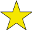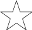## Pillow-Problems: #27

Difficulty:(explanation of difficulty)

The book Pillow-Problems: Thought Out During Wakeful Hours, by Charles Dodgson, better known by the pseudonym Lewis Carroll, was first published in 1893. It contains 72 problems that Carroll thought of while lying awake at night over the course of a few decades. Carroll's intent was that the puzzler would, like he did, work out the answers to the questions mentally. This is problem #27 in the book.
Previous problem: .
Next problem: .

### 27.

There are 3 bags, each containing 6 counters; one contains 5 white and one black; another, 4 white and 2 black; the third, 3 white and 3 black. From two of the bags (it is not known which) 2 counters are drawn, and prove to be black and white. What is the chance of drawing a white counter from the remaining bag?

[4/3/80

Call the bags A, B, C.

if remaining bag be A, chance of observed event = ½ chance of drawing white from B and black from C + ½ chance of drawing black from B and white from C:

i.e. it = ½ · {

 (2)/ (3)
× ½ +
 (1)/ (3)
× ½} = ¼.

Similarly, if remaining bag be B, it is ½ · {

 (5)/ (6)
· ½ +
 (1)/ (6)
· ½} = ¼ and, if it be C, it is ½ · {
 (5)/ (6)
·
 (1)/ (3)
+
 (1)/ (6)
·
 (2)/ (3)
} =
 (7)/ (36)
.

∴ chances of remaining bag being A, B, or C, are as ¼ to ¼ to

 (7)/ (36)
; i.e. as 9 to 9 to 7. ∴ they are, in value,
 (9, 9, 7)/ (25)
.

Now, if remaining bag be A, chance of drawing white from it is

 (5)/ (6)
; ∴ chance, on this issue, is
 (5)/ (6)
·
 (9)/ (25)
=
 (3)/ (10)
; similarly, for B, it is
 (2)/ (3)
·
 (9)/ (25)
=
 (6)/ (25)
; and, for C, ½ ·
 (7)/ (25)
=
 (7)/ (50)
. And either chance of drawing white from the remaining bag is the sum of these; i.e.
 (15 + 12 + 7)/ (50)
=
 (34)/ (50)
=
 (17)/ (25)
.